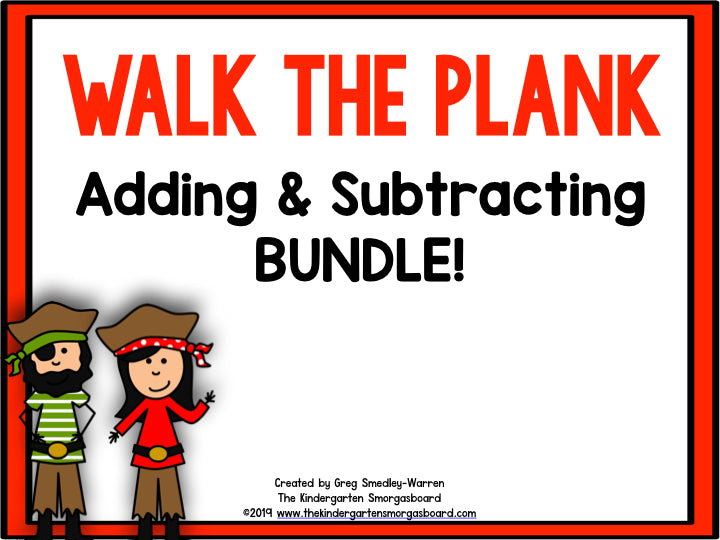Regular price \$18.00 \$13.50 Sale

This BUNDLE is designed to help students practice and master their addition & subtraction skills. The activities in this creation can be differentiated and used in a variety of ways to meet the needs of all students. Most of the activities include recording sheets that can be used for student accountability.

Includes:

• Subtraction puzzles
• Story problems
• Subtraction sort
• Subtract and Clip
• Missing numbers
• Missing Puzzles

This BUNDLE includes 2 creations and is discounted by 25% off of the regular price.  The creations included are:

Pirate Subtraction!

Centers Included:

• Differentiated Walk, Subtract and Write The Room
• Subtraction sort
• Subtraction puzzles
• Story problems
• Missing Numbers
• Subtraction Circle Maps
• Subtract and Clip